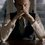# Another Missouri state problem!

The first two parts build the tempo. Once you solve these two(please don't see the solution), move to the last part.

If six numbers are chosen at random, uniformly and independently, from the interval [0,1], what is the probability that they are the lengths of the edges of a tetrahedron?

My attempt -

Once I have chosen such $x,y,z$ which form a triangle, number of points lying inside that triangle will be proportional to the number of tetrahedrons.

So, the $\text{Probability(x,y,z, a,b,c forming a tetrahedron | x,y,z form a triangle)} \propto \text{Area of the triangle}$

$= \frac{k}{4} \sqrt{(x+y+z) (x+y-z) (x-y+z) (-x+y+z)}$

$= \iiint \frac{k}{4} \sqrt{(x+y+z) (x+y-z) (x-y+z) (-x+y+z)} \ dx \ dy \ dz$

$\displaystyle = Re\left( \int_{z=0}^{z=1} {\int_{y=0}^{y=1}{\int_{x=0}^{x=1}{ \frac{k}{4} \sqrt{(x+y+z) (x+y-z) (x-y+z) (-x+y+z)} \ dx} \ dy }\ dz} \right)$

I don't really know how to follow next, or if what I did until now is any helpful or not.Note by Kartik Sharma
4 years, 4 months ago

This discussion board is a place to discuss our Daily Challenges and the math and science related to those challenges. Explanations are more than just a solution — they should explain the steps and thinking strategies that you used to obtain the solution. Comments should further the discussion of math and science.

When posting on Brilliant:

• Use the emojis to react to an explanation, whether you're congratulating a job well done , or just really confused .
• Ask specific questions about the challenge or the steps in somebody's explanation. Well-posed questions can add a lot to the discussion, but posting "I don't understand!" doesn't help anyone.
• Try to contribute something new to the discussion, whether it is an extension, generalization or other idea related to the challenge.

MarkdownAppears as
*italics* or _italics_ italics
**bold** or __bold__ bold
- bulleted- list
• bulleted
• list
1. numbered2. list
1. numbered
2. list
Note: you must add a full line of space before and after lists for them to show up correctly
paragraph 1paragraph 2

paragraph 1

paragraph 2

[example link](https://brilliant.org)example link
> This is a quote
This is a quote
    # I indented these lines
# 4 spaces, and now they show
# up as a code block.

print "hello world"
# I indented these lines
# 4 spaces, and now they show
# up as a code block.

print "hello world"
MathAppears as
Remember to wrap math in $$ ... $$ or $ ... $ to ensure proper formatting.
2 \times 3 $2 \times 3$
2^{34} $2^{34}$
a_{i-1} $a_{i-1}$
\frac{2}{3} $\frac{2}{3}$
\sqrt{2} $\sqrt{2}$
\sum_{i=1}^3 $\sum_{i=1}^3$
\sin \theta $\sin \theta$
\boxed{123} $\boxed{123}$

Sort by:

This is a complicated matter. The third vertex of a tetrahedron does not have to lie above the base. For that matter, the uniform distribution of the side lengths will not convert into a uniform distribution of the (projected) location of the fourth vertex onto the base.

You might like to look at the article by Wirth & Dreiding that can be found here.

- 4 years, 4 months ago

Oh I see. It's really a complicated matter. And indeed out of my abilities as well.

- 4 years, 4 months ago